×

Search anything:

# Examples of Hash Functions

#### Algorithms HashingGet this book -> Problems on Array: For Interviews and Competitive Programming

In this article, we have listed several examples of good Hash Functions which you are used conveniently. This can be used to hash any data (numeric and string). Some examples are PJW hash, Division Hash, BUZ hash and much more.

1. Introduction to Hash Function
2. Division Hash
3. Knuth Variant on Division Hash
4. Multiplication Hashing
5. Hashing functions for strings
• PJW hash
• CRC variant of hashing
• BUZ hash
6. Universal Hashing
7. Perfect Hashing
8. Question
9. References

### Introduction to Hash Function

As hash tables if used properly are a boon to the human community as they save a lot of time in searching important data, it is of utmost iportance that we should know how to use hash tables for our benefits.

The amount by which how better hash tables function depend a lot on the type of hash function being used to generate corresponding value of key, we should analyse some popular hash functions and some techniques by which we can make our hash tables less resource consuming.

Qualitative information about the keys can be useful in the designing of good hashing functions.

An ideal hash function maps keys to hash values randomly, that is every bucket is equalll fulfilled so as to keep the average complexity as low as possible, even though there are some patterns in the input, and mapping of one key does not depend upon the mapping of oher keys, which is also called as simple uniform hashing.

The different examples of Hash Functions are:

• Division Hash
• Knuth Variant on Division Hash
• Multiplication Hashing
• Hashing functions for strings
• PJW hash
• CRC variant of hashing
• BUZ hash
• Universal Hashing
• Perfect Hashing

## 1. Division Hash

Probably most common type of hash function to ever exist on this planet. It uses basic poperties of division to generate the values for the corresponding keys.

Function:-
h(k)=k mod m
where k is the key and m is the size of our hash table.We should choose size whoch is a prime and not close to a power of 2. It does not work as desired if there are some patterns in the input data.
Example:-
If k is 44 and m is 13, then h(k)=5, 44 divided by 13 gives remainder 5.
Therefore, this key k=44 will be mapped to slot number 5.

## 2. Knuth Variant on Division Hash

It is slightly different than normal division hash function.
Somehow it works better than the raw division method.

Function:-
Here, h(k)=k(k+3) mod m,
with m being the size of the hash table and k being the key, with h(k) being the hash value.
Example:-
Suppose our key k is 1 and m is 13, then, h(1)=1(1+3) mod 13
=4 mod 13
=4.
Therefore, this key k=1 will be mapped to slot number 4.

## 3. Multiplication Hashing

First, we multiply the key k by a constant A in the range 0 < A < 1 and extract the fractional part of kA. Then, we multiply this value by m and take the ﬂoor of the result.

Function:-
In short, the hash function is
s = kA
x = fractional part of s
h(k) = floor(mx)

This method works for any value of A, it works better for some values than with others.

According to Knuth A should be equal to the value of golden ratio(A=(root(5)-1)/2).
In this type of hash function the value of m is not critical, therefore we choose m to be some power of 2,(m=2^p, for some integer p), so that this function can be easily calculated on computers.

Take A to be s/2^w where w is the word size of the computer, and s is the integer in the range 0<s<2^w, and relatively prime to 2^w.Multiply K by s to obtain a 2w-bit product in which the lower w bits contain the fractional part of the product , that is, Now take the table size m to be 2^p for some integer p; then h(K) is simply the leading p high order bits of fractional part of the product. To retrieve h(K), set all bits to the left of the imaginary radix point to 0 and then shift right p bits.
Those p-bits extracted are the value of h(k).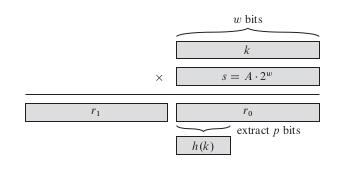Example:-

If we take k = 246912, p = 14, m = 2^14= 16384,and w = 32. Adapting Knuth’s suggestion, we choose A to be the fraction of the form s=2^32 that is closest to (root(5)- 1)/2, so that A=2654435769/2^32 . Then, k  s =327706022297664=(246912  2^32) + 35225728 , and so r1=246912

and r0 =35225728. The 14 most signiﬁcant bits of r0 yield the value h(k)=134.

# Hashing functions for strings

Basically, strings are array of characters and tables are indexed numerically, therefore we need to design a hashing function which output some numeric value.
A lot of attention has been put into the design of hash function for strings whch can be easy to compute for strings and distributes strings uniformly among the m(size of the table) lists.
One approach of computing hash functions for the strings is to convert the characters of the strings into integers k1,...,kn with n being the length of the string, conversion should be according to ASCII values, and they must be unsigned. To start asssign h(hash value) to be equal to zero(for 1st iteration in the loop), basic difference among these algorithms is how these integers are combined with the value of h(i.e. the output value).

## 1. PJW hash

It was developed by Peter J. Weinberger of AT&T labs.

Algorithm:-

``````int fun(char *p)
{
while(*p!=NULL)
{
h=(h<<4)+(*p);
if(g=h & 0xf0000000)
{
h=h XOR (g>>24);
h=h XOR g;
}
}
return h % m;
}
``````

Here, ">>" means righ shift, "<<" means left shift and "&" is a bitwise AND operator.
Here, m is the size of the table and should be prime.

1.Begin with the value of h=0.
2.Do bitwise left shift operation over h, and left shift it by 4 bits and add that value to the binary value of the current character, and equate that value to h.
3. In the if condition do a bitwise AND operation between h and 0xf0000000,and equate that value to a variable g, if the computed value is not equal to zero, then enter into the condition and XOR the value of h with 24 bits right shifted value of h, and then equate it to h.
4.In the same if condition if XOR the values of h and g, and again equate it with h.
5.If the if condition was not satisfied in the first place then skip that condition and just loop to the next character.
6.Repeat the above steps till you reach the NULL character.
7.Use the value of h after the loop has ended and divide it by m to take the remainder and output that value as the hash value for that key.

## 2. CRC variant of hashing

CRC basically stands for Cyclic Redundancy Check, and is generally used to check errors in the data which are transmitted through any lossy medium like ethernet, since it converts an input of strings into a smaller output value they can also be used as a hash function.
It generally works better than PJW hash.

Algorithm:-

``````int fun(char* p)
{
while(*p!=NULL)
{
highorder = h & 0xf8000000;
h = h << 5;
h = h XOR (highorder >> 27);
h = h XOR *p;
}
return h % m;
}
``````

Here, m is the size of the hash table and should be prime, with "<<" meaning left shift, ">>" meaning right shift, and "&" is bitwise AND operator, with "ki" being the ith character of the string.

*1.Begin with the value of h=0.
2.extract high-order 5 bits from h and 0xf8000000(which is the hexadecimal representation for the 32-bit number), and equate it to variable highorder.
3.shift h left by 5 bits.
4.Do 27 bits right shift operation over highorder and XOR the value with h and equate the resulted value to h.
5.XOR the value of h with character's binary value and equate it with h.
6.Repeat the above steps till you reach the NULL character.
7.Use the value of h after the loop has ended and divide it by m to take the remainder and output that value as the hash value for that key.
*

## 3. BUZ hash

It involves a random number generator which can be stored in array,which can be run to produce an array of randomly generated values, and then steps can be done as in the algorithm to generate the hash value for the string.

Algorithm:-

``````int fun(char* p)
{
while(*p!=NULL)
{
highorder = h & 0x80000000 ;
h = h << 1 ;
h = h XOR (highorder >> 31);
h = h XOR R[*p] ;
}
return h % m;
}
``````

Here, m is the size of the hash table and should be prime, with "<<" meaning left shift, ">>" meaning right shift, and "&" is bitwise AND operator, also "ki" is the ith character of the string.

1.Begin with the value of h=0, and a random number generator generating a random number for each character for the string and storing it in an array with the index equal to the position of that character in the string.
2.Do the bitwise and operation between h and 0x80000000, and equate that value to a variable named highorder.
4.Left shift h by 1 unit towards left, and store that value into h.
5.Do the bitwise right shift operation over high order by 31 places, and XOR that value by h and store it into h.
6.From the array generated by random function, XOR the value of h and the random number generated from random number generated stored at the index equal to that character's index in the array.
7.Repeat the above steps till you reach the NULL character.
8.Use the value of h after the loop has ended and divide it by m to take the remainder and output that value as the hash value for that key.

Probability of collision for all the above algorithms:-

In all the above algorithms(hash for numbers to hashing for strings) since, we have assumed simple uniform hashing i.e. keys are random and we are applying the above mentioned hash functions to them, suppose there are "p" keys and "n" is the number of slots. Then, probability of no collision for random input is:-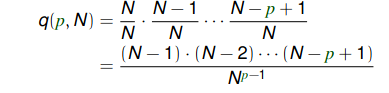## Universal Hashing

Let us dive deep into the more fascinating hashing functions which almost every time guarantee that the time complexity of search, insert and delete is constant expected time(in reality it is θ(1+α)).

Basically, what we were asuming till now was that our hashing functions were fixed which if known by the malicious attackers can create an input which may cause the placement of all the keys in the same slot, which may have various ramifications depending upon the context.

What we are going to do in Universal Hashing is that we will have a class of hash functions satisfying a particular property, that the probability of two keys colliding for the hash function belonging to that universal class of hash function must be less than or equal to 1/(size of the hash table), and for each time the program is run the hashing function which is going to work on the input will be randomly choosen from that class of hashing functions and thus, depriving the attackers from creating any such input values which can be disastrous to the system, as no same input can evoke the worst case twice, due to the property of universal hashing function.

One example of universal hash family is given below:-
Dot product hash family:-

1. Assuming m is prime.
2. Assuming the total number of keys, let it be u, u=m^r(for some integer r), if m^(p-1)<u<m^p, r =p.
3. View key k in base m.
k=<k0,k1,...,kr-1> with each of 0<=ki's<(m-1), with ki being the (i+1)th digit of key k in base m.
4.For key a=<a0,a1,a2,a3,...,ar-1>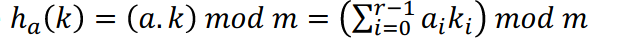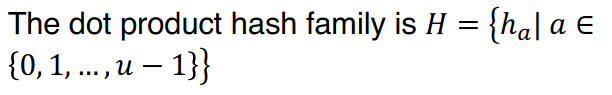**** Probability of collision:-****

Here, the collision proability will be at most equal to (1/m).

## Perfect Hashing

Let us discuss about the cooler version of hash function that is perfect hashing in which the search time is constant for the static set of the data, means we forbid the insert and delete operation.
Perfect hashing is also known as FKS hashing as it was developed by Fredman, Komlos, and Szemeradi.

1. O(1) worst case time for search.
2. O(n) worst case space.
3. Polynomial build time with high probability.
It may take a little bit of time to build the data structure but once developed we are gonna have perfect scenario for our search.
We don't want to store the set of colliding elements in a linked list as linked lists are slow therefore, we will use two levels of hashing in the case of perfect hashing, we will be using hash tables to store those elements which collide with each other.Hash tables will vary in sizes and some of them will be of size 0, as nothing is colliding there.
If total number of keys colliding at any slot is l, then in the second level hashing there would be l^2 no. of slots in the hash table, we use l^2 as it is immediately derived from the birthday paradox which states (as if there are n people, with n^2 possible birthdays ,then the probability of getting two people whose birthday collide is 1/2).
But, 1/2 is not that great therefore, we will be using some another trick so that they don't collide at all.
Steps:-
4. Pick h1(hash function at level 1) from the universal class of hash function.
For each slot j belonging to any of the m slots, lj is the number of keys hashing to the same slot, m= theta(n).
5. If summation of l^2 > c.n, then redo 1.
3.Pick h(2,j)(hashing function for secondary hash table for slot j(occurring in the first level)) from same universal class of hash function with the size of hash function being l^2.
6. If h(2,j)(ki)=h(2,j)(ki') for some j, ki not equal to ki', and h1(ki) being equal to h1(ki'), redo step 3.
Just continue randomly picking those hash functions, till there is no collision.
After this step there would be no collisions, with proper notion of the word collision that is two different keys mapping to the same value. Guaranteed constant time search after all these steps.
We will have to redo these steps at most of O(n(log n)^2) times before we can have our desired data structure for constant time search.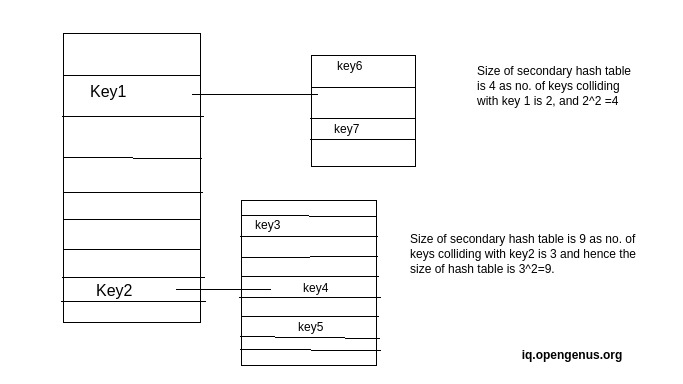Probability of collision:-
Collision probabiity in this case is 0, as our data structure is designed in a way that there is no collision in it.

## Using which technique, can we hope to get the searched item in constant time.

#### the question text

Perfect Hashing
Universal hash
Multiplicative method for hashing.
PJW hash
As perfect hashing search time complexity is constant once the data structure is built.
1. Thomas H. Cormen, Charles E. Leiserson, and Ronald L. Rivest (1990) Introduction to Algorithms, MIT Press, Cambridge MA and McGraw-Hill, New York.
2. Data Structures by Evangel P.Quiwa.
3. Alfred V. Aho, Ravi Sethi, and Jeffrey D. Ullman, Compilers: Principles, Techniques, and Tools, Addison-Wesley, 1986.